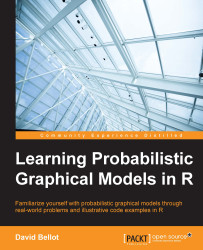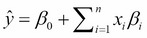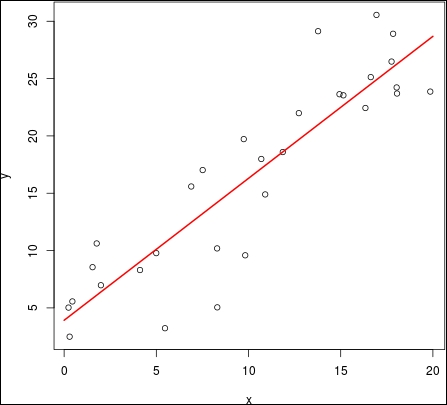•#### Learning Probabilistic Graphical Models in R#### Overview of this book

Probabilistic graphical models (PGM, also known as graphical models) are a marriage between probability theory and graph theory. Generally, PGMs use a graph-based representation. Two branches of graphical representations of distributions are commonly used, namely Bayesian networks and Markov networks. R has many packages to implement graphical models. We’ll start by showing you how to transform a classical statistical model into a modern PGM and then look at how to do exact inference in graphical models. Proceeding, we’ll introduce you to many modern R packages that will help you to perform inference on the models. We will then run a Bayesian linear regression and you’ll see the advantage of going probabilistic when you want to do prediction. Next, you’ll master using R packages and implementing its techniques. Finally, you’ll be presented with machine learning applications that have a direct impact in many fields. Here, we’ll cover clustering and the discovery of hidden information in big data, as well as two important methods, PCA and ICA, to reduce the size of big problems.
Learning Probabilistic Graphical Models in RCreditswww.PacktPub.comPrefaceFree Chapter
Probabilistic ReasoningExact InferenceLearning ParametersBayesian Modeling – Basic ModelsApproximate InferenceBayesian Modeling – Linear ModelsProbabilistic Mixture ModelsAppendixIndex## Linear regression

We start by looking at the most simple and most used model in statistics, which consists of fitting a straight line to a dataset. We assume we have a data set of pairs (xi, yi) that are i.i.d and we want to fit a model such that:

y = βx +β0 + ϵ

Here, ϵ is a Gaussian noise. If we assume that xi ϵ n then the expected value can also be written as:Or, in matrix notation, we can also include the intercept β0 into the vector of parameters and add a column on 1 in X, such that X = (1, x1, …, xn) to finally obtain:

ŷ = XTβ

The following figure shows an example (in one dimension) of a data set with its corresponding regression line:In R, fitting a linear model is an easy task, as we will see now. Here, we produce a small data set with an artificial number, in order to reproduce the previous figure. In R, the function to fit a linear model is `lm()` and it is the workhorse of this language in many situations. Of course, later in this chapter we will see more advanced algorithms:

`N=30...`